# HSPT Math : Arithmetic

## Example Questions

### Example Question #51 : Concepts

Fill in the blank with the proper sign.__________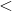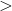Explanation:

In order to solve this problem, we will use a number line. Numbers to the left of zero on the line are less than numbers to the right.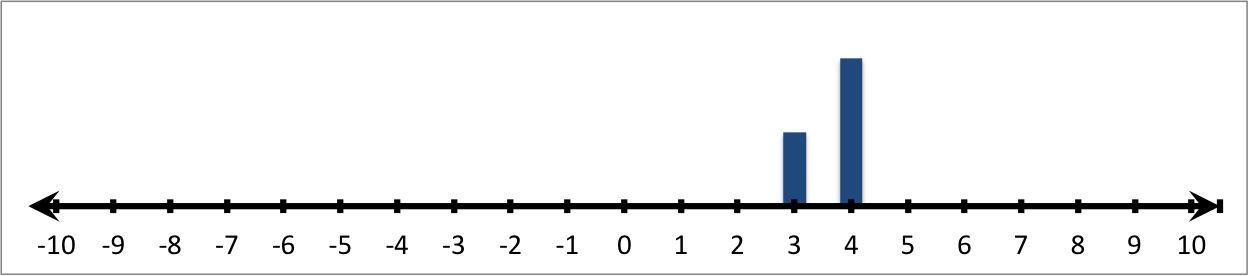Therefore: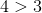### Example Question #11 : Understand An Inequality On A Number Line: Ccss.Math.Content.6.Ns.C.7a

Fill in the blank with the proper sign.__________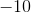Explanation:

In order to solve this problem, we will use a number line. Numbers to the left of zero on the line are less than numbers to the right.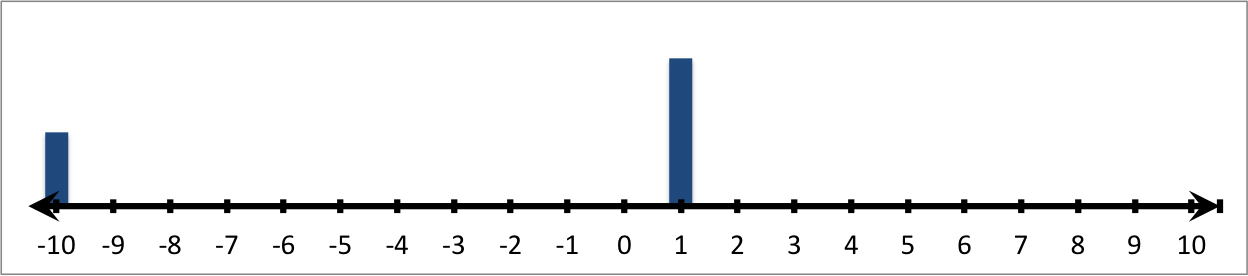Therefore: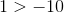### Example Question #51 : Concepts

Fill in the blank with the proper sign.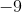__________Explanation:

In order to solve this problem, we will use a number line. Numbers to the left of zero on the line are less than numbers to the right.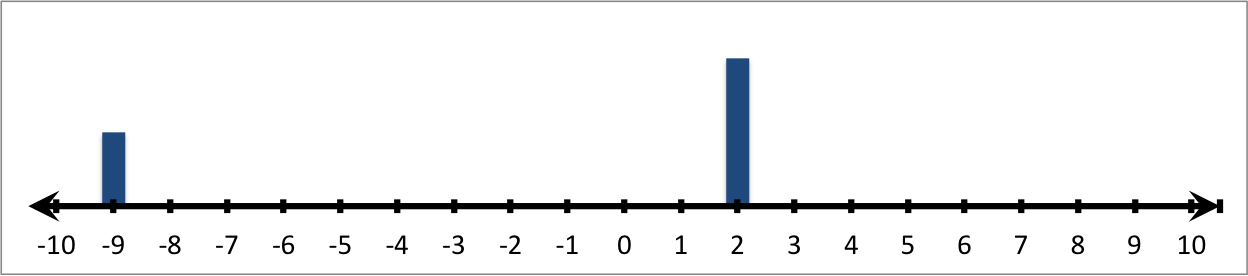Therefore: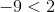### Example Question #51 : Negative Numbers

Fill in the blank with the proper sign.__________Explanation:

In order to solve this problem, we will use a number line. Numbers to the left of zero on the line are less than numbers to the right.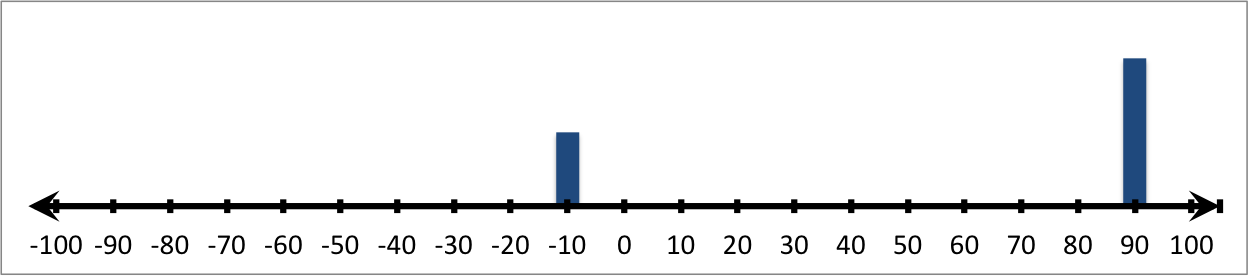Therefore: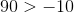### Example Question #52 : Concepts

Fill in the blank with the proper sign.__________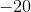Explanation:

In order to solve this problem, we will use a number line. Numbers to the left of zero on the line are less than numbers to the right.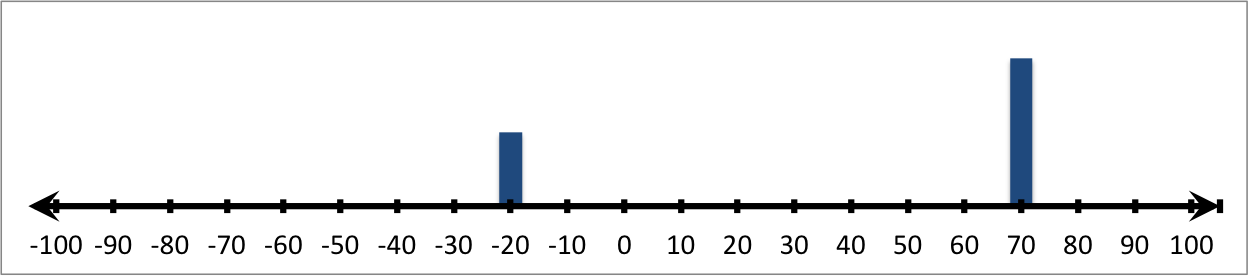Therefore: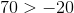### Example Question #53 : Concepts

Fill in the blank with the proper sign.__________Explanation:

In order to solve this problem, we will use a number line. Numbers to the left of zero on the line are less than numbers to the right.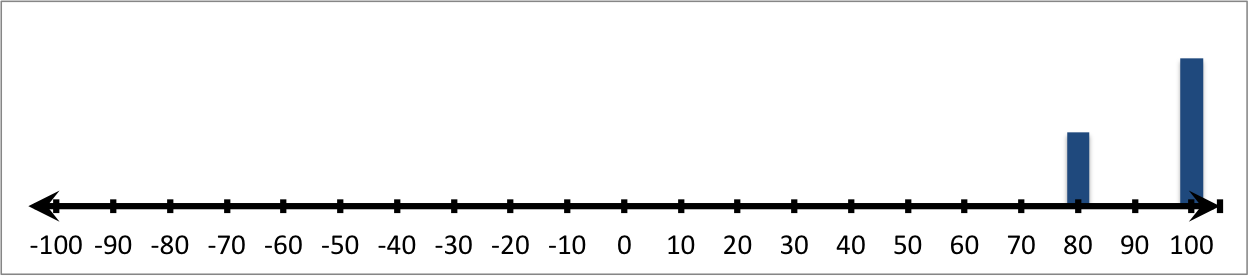Therefore: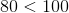### Example Question #11 : Understand An Inequality On A Number Line: Ccss.Math.Content.6.Ns.C.7a

Fill in the blank with the proper sign.__________Explanation:

In order to solve this problem, we will use a number line. Numbers to the left of zero on the line are less than numbers to the right.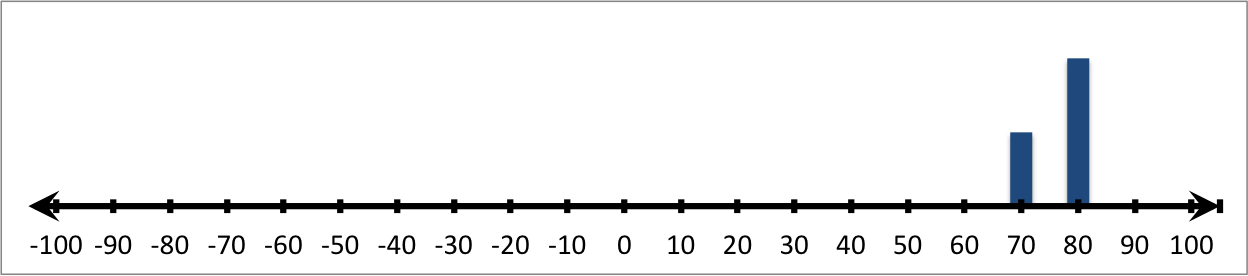Therefore: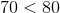### Example Question #51 : Concepts

Fill in the blank with the proper sign.__________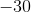Explanation:

In order to solve this problem, we will use a number line. Numbers to the left of zero on the line are less than numbers to the right.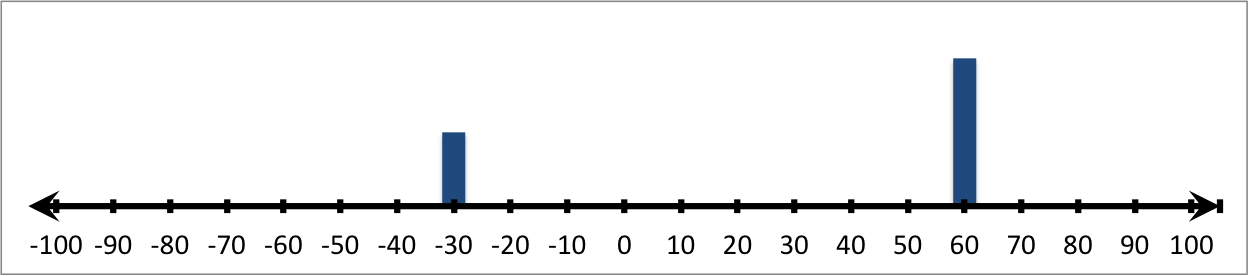Therefore: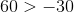### Example Question #471 : The Number System

Fill in the blank with the proper sign.__________Explanation:

In order to solve this problem, we will use a number line. Numbers to the left of zero on the line are less than numbers to the right.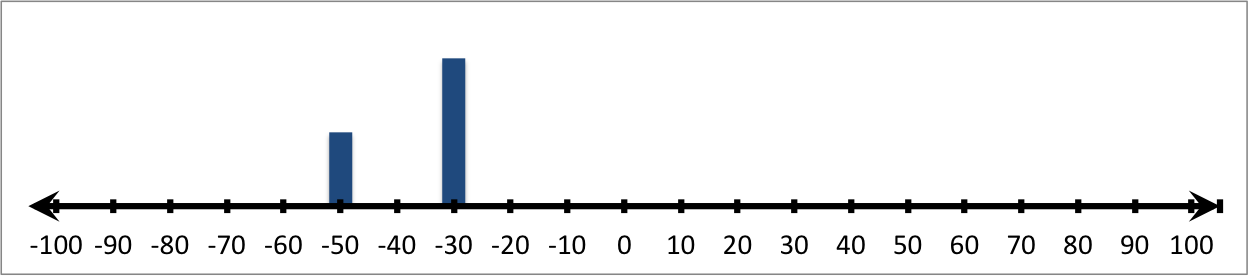Therefore:### Example Question #11 : Understand An Inequality On A Number Line: Ccss.Math.Content.6.Ns.C.7a

Fill in the blank with the proper sign.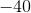__________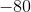Explanation:

In order to solve this problem, we will use a number line. Numbers to the left of zero on the line are less than numbers to the right.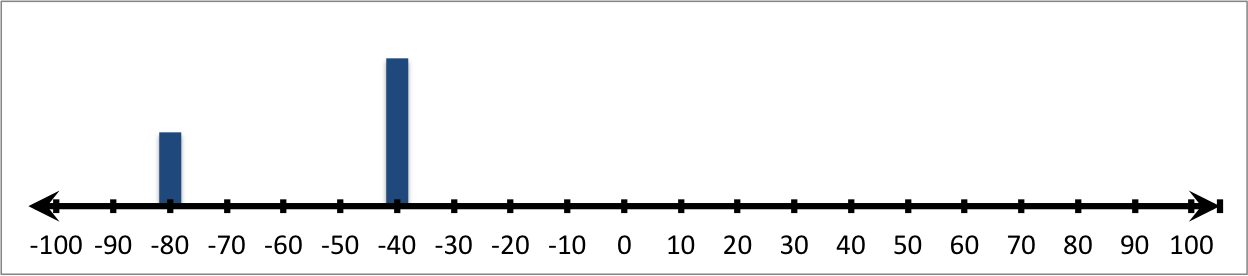Therefore: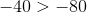### All HSPT Math Resources